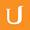## Joint Variation Practice 2 - Visualizing Algebra

• 0:00 - 0:06
it turns out the value of X would be 84 when Y is & and Z is 1. Nice work if you
• 0:06 - 0:10
figured that one out. We know the values for X1, Y1, and Z1 so we plug those in
• 0:10 - 0:16
here. We are looking for the X value when Y is 7 and Z is 1, so we plug in 7 and
• 0:16 - 0:21
1 in these positions. We can think of these numbers as being the second case
• 0:21 - 0:27
We're looking for x 2, which is x, and we know the second y value is 7, and the
• 0:27 - 0:32
second z value is 1. We use these lists to make our substitution. We simplify
• 0:32 - 0:37
this right hand side to get 5 42nds. Next we multiply both sides of the equation
• 0:37 - 0:42
by the LCD, 42x. Essentially, this is the same as cross-multiplying. So we get
• 0:42 - 0:49
5x on the right and 420 on the left. We divide both sides by 5 to get x is equal
• 0:49 - 0:49
to 84.
Cím:
Joint Variation Practice 2 - Visualizing Algebra
Video Language:
English
Team:Udacity
Projekt:
MA006 - Visualizing Algebra
Duration:
0:50Udacity Robot edited Angol subtitles for Joint Variation Practice 2 - Visualizing AlgebraCogi-Admin hozzáadott egy fordítást

# English subtitles

## Felülvizsgálatok Compare revisions

• API
Udacity Robot